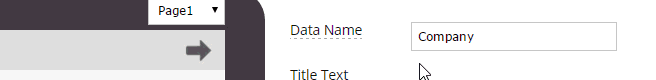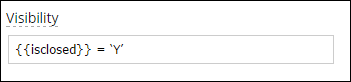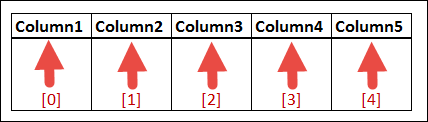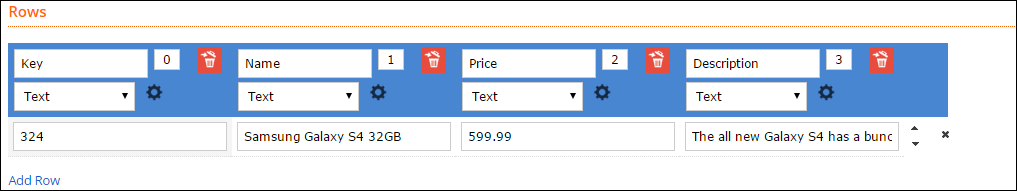Most advanced Form functions involve the use of a formula.
As such it is critical that you understand how to create formulae in order to get the most out of our advanced features.

First off you need to know how to address or refer to a field in your Form.

This is done by using
placeholders wherever you want the system to insert answers from fields of your Form.
Placeholders are entered using the unique data name property of the Form field, wrapped in curly braces:  {{dataname}}So for example if you have a Page group called "page1" and a Text field named "xyz" inside that group:

• You would refer to xyz as:  {{xyz}}
• Refer to page1 with:  {{page1}}

Note that placeholders and all other functions available for use in a formula are case sensitive.

Now that you know how to reference field values, you can use this knowledge to build a formula.
A formula contains placeholders and/or various operators, and functions, which together give a result - much the same as an Excel formula works.

When the app runs the formula, any placeholders are replaced with the value currently stored in the field referenced by that placeholder.

Let's look at a simple example.

Say we have a question that asks whether a road has been closed (field's data name is "isclosed"), and a follow-up question that asks what the reason for the closure is (field's data name is "closedreason").

The "isclosed" field has two options: Yes (underlying value Y) or No (underlying value N).If the user answers No, then we don’t want to show the "closedreason" field.

This calls for a Visibility condition on the "closedreason" question, defined as a formula: {{isclosed}} = ‘Y’ What the above formula says is that the "closedreason" question is only Visible if "isclosed" was answered with the Y (Yes) value.

Note that we use single quotes ( ' ) to include literal/fixed values - i.e. the 'Y' part of the formula.Let’s look at a more advanced constraint example.
Say we want have a "dateofbirth" question that we want to constrain to only allow dates in the past.

In this case, we would use a function in our formula to evaluate today’s date:

{{dateofbirth}} <= today()

The function "today()" is one of many functions available for use in your formula.

Referring to column values of a selected row from a Data Source

The Form Designer allows you to link Choices fields to a Data Source that you have previously uploaded.

Data Sources can have as many columns as you like, and you may want to refer to the values in these columns when creating a Form.
To do so you will need to use a formula to refer to the desired column.

By default the first column in a Data Source (also known as the Value column) is always used as a field's answer.
If you want to refer to any other column's value, you need to use an index syntax - specifically you need to add square brackets around the column's index number - e.g. {{product[index]}}

Data Source column indexes always start at zero - i.e. the first column has index of 0, the second column has index 1, third column is index 2, and so on.Lets look at an example
Imagine you have a Data Source called "Products" that contains your product list, with rows such as:You create a Form named "Sales Order" which you will use to capture orders for your products.

The Form has the following fields:

• product_choice : A Choices field that is linked to the Products Data Source.
• quantity : A Number field that captures the quantity of product ordered.
• total : A Number field that will calculate the total amount by multiplying the product's price by quantity.

On the "total" field, you would define a Dynamic Value formula as follows: {{product_choice}} * {{quantity}}Note the square brackets and index value of 2 used to refer to the 3rd column (the Price) in the Products Data Source.